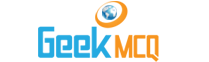Engineering :: Industrial Engineering

1.  At 'break even point'
 A. Constant expenses = Profits B. Total sales = variable expenses C. Variable expenses - Profits = Total sales D. None of the above

2.  In a break even chart, abscissa represents
 A. Constant expenses B. Total expenses C. Profits D. Total sales E. Amount in rupee

3.  By technological innovations if the cost of raw material is reduced, this will result in
 A. Increases constant expenses B. Reduced constant expenses C. Lower profits D. Lower break even point E. High break even point

4.  Total sales less cost of manufacturing gives
 A. Net B. Gross profit C. Distributable profit D. Taxable profit E. Book profit

5.  In motion and time study which of the following is used in product analysis?
 A. Process chart B. Work place layout C. Man operation chart D. Multi-man process chart E. All of the above

6.  In motion and time study which of the following is used in man analysis?
 A. Man and machine analysis chart B. Man and maching operation time chart C. Man and machine, process time chart D. Multiman operation time chart E. All of the above

7.  While selecting site for a regional paint, which factory services (is) are to be looked into?
 A. Adequacy of supply of power B. Water supply C. Sewage disposal system D. Availability of manpower E. All of the above

8.  The advantage of product layout is
 A. Lower total materials handling cost B. Lower total production time C. Less work-in-process D. Less floor area required per unit of production E. All of the above

9.  The advantage of process layout is
 A. Less duplication of equipment B. Greater flexibility of production C. Better control of complicated or precision processes D. Easier to handle breakdown of equipment E. All of the above

10.  In time and motion study, the symbol 'O' represents
 A. Quantity inspection B. Quality inspection C. Temporary storage D. Obvious delay E. Operation